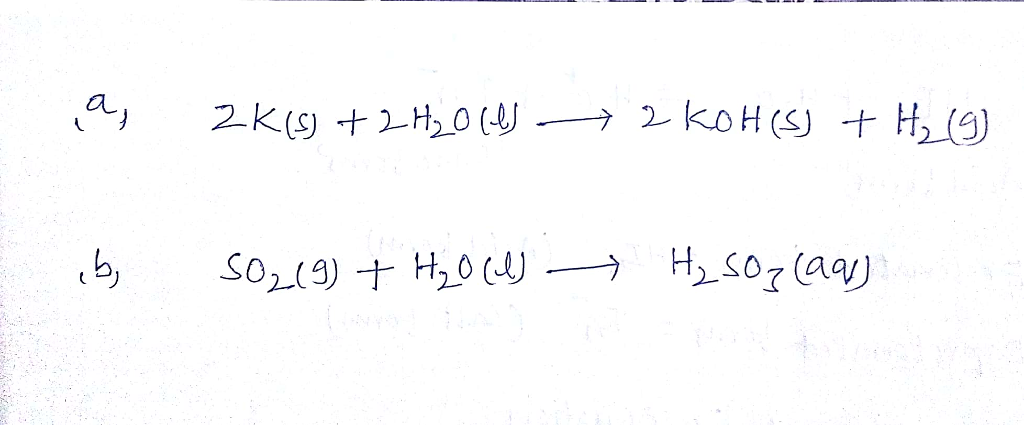# Question & Answer: Write a chemical equation for the following descriptions: (Use the lowest possible coefficients…..

Write a chemical equation for the following descriptions: (Use the lowest possible coefficients. Use the pull-down boxes to specify states such as (aq) or (s). If a box is not needed, leave it blank.) (a) Two atoms of potassium metal react with two molecules of liquid water to produce two molecules of solid potassium hydroxide (KOH) and one molecule of hydrogen gas. (b) One molecule of sulfur dioxide gas reacts with one molecule of liquid water to produce one molecule of liquid sulfurous acid (H_2SO_3).